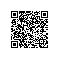# java产生随机数的方法

1. java.lang.Math.random()

1. Math.random() * 10

1. Math.round(Math.random() * 10)

1. new java.util.Random().nextInt(10)

Chris Marasti-Georg 指出:

1. Math.round(Math.random() * 10)

1. Math.floor(Math.random() * 11）

Java实战项目里面介绍了一些不正确使用java.util.Random API的危害。这个教训告诉我们不要使用：

1. Math.abs(rnd.nextInt())%n

1. rnd.nextInt(n)使用钉钉扫一扫加入圈子
+ 订阅